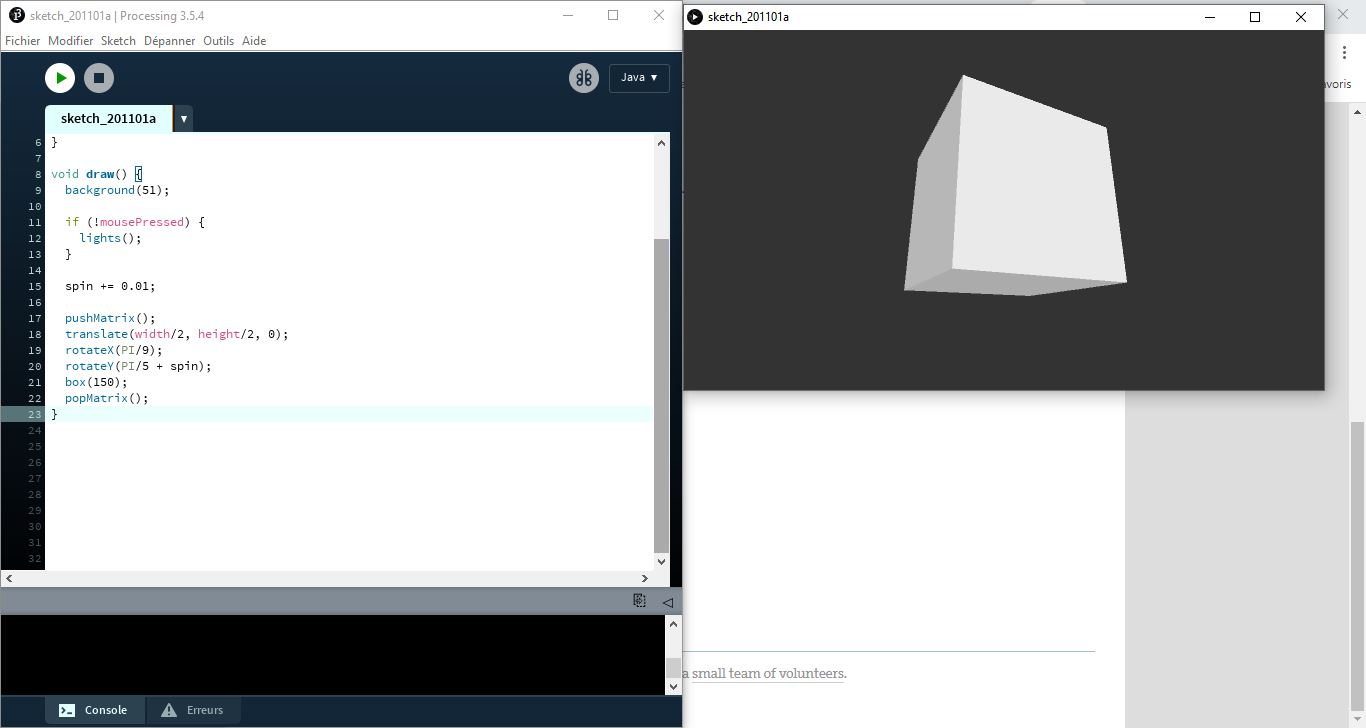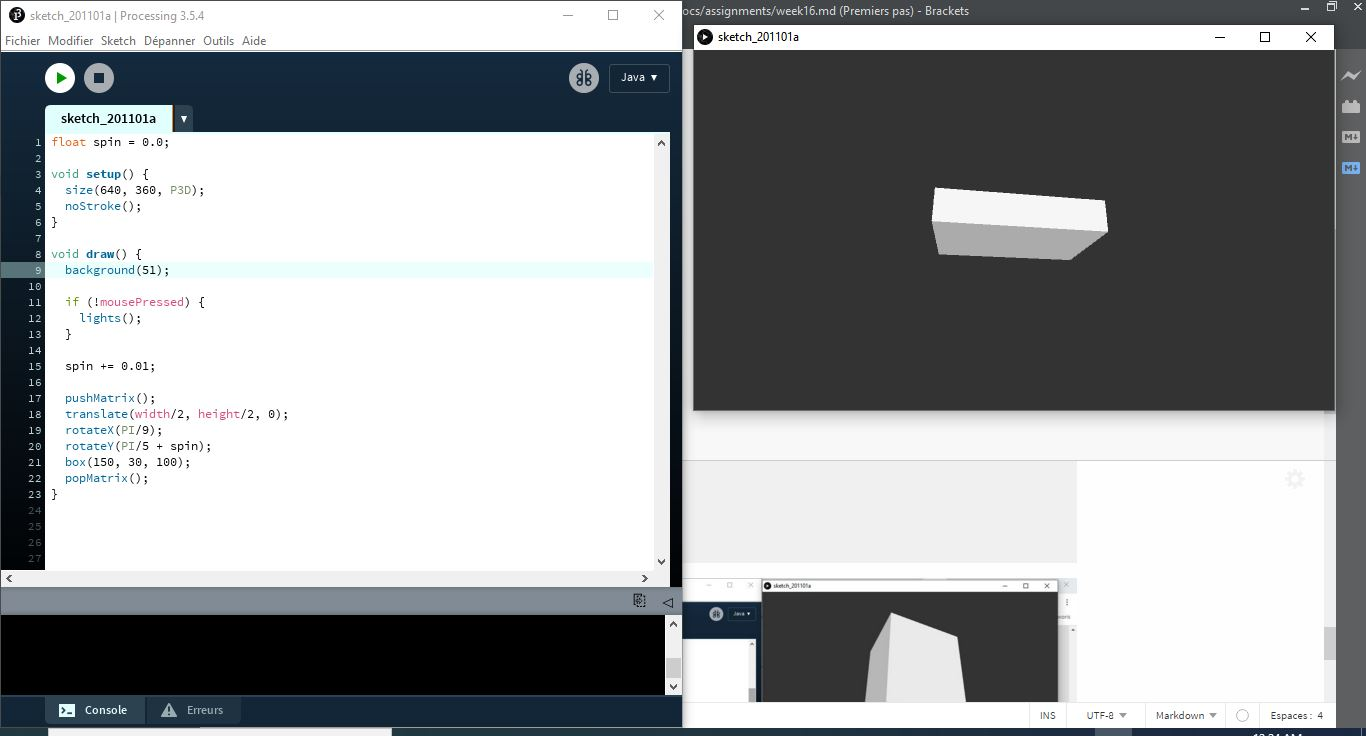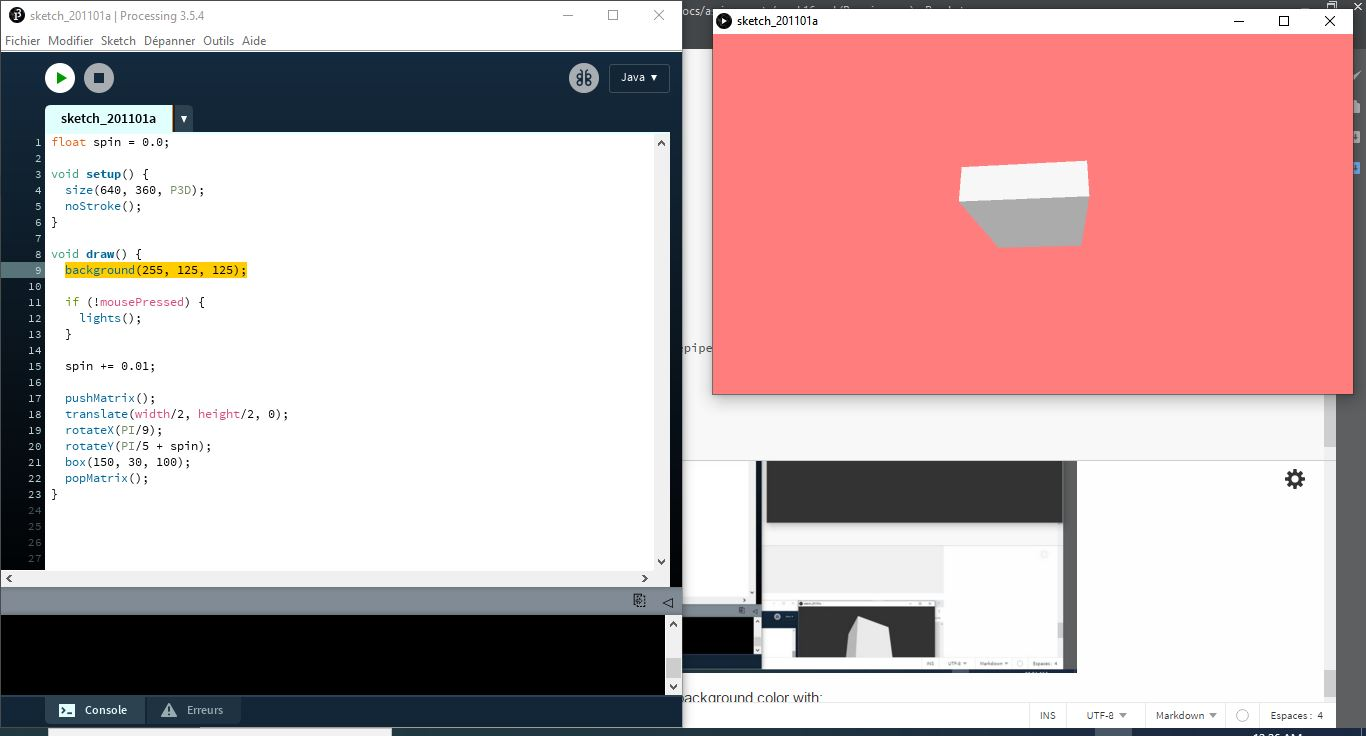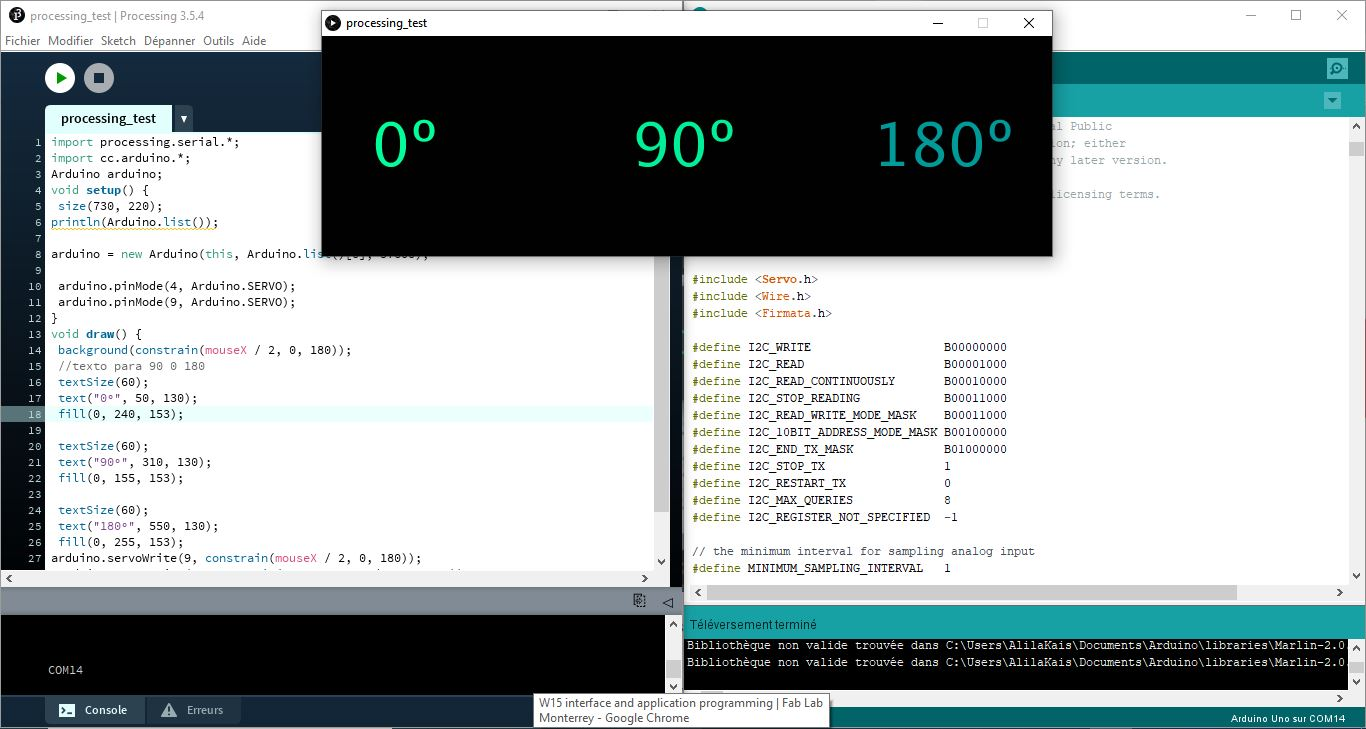# 16. Interface and application programming¶

## Individual assignment¶

This week we had to write or modify an application that interfaces with a device.

As I have never writed an application before so first I wanted to get introduced to Processing.

What is Processing?

Processing is a flexible software sketchbook and a language for learning how to code within the context of the visual arts. Since 2001, Processing has promoted software literacy within the visual arts and visual literacy within technology. There are tens of thousands of students, artists, designers, researchers, and hobbyists who use Processing for learning and prototyping.

## Getting started¶

To gett strated with processing I uploaded some of the examples and modify them to see the results. First I used OnOff sketch and modify color, shape and movement making adjustments to the parameters in the code.

``````float spin = 0.0;

void setup() {
size(640, 360, P3D);
noStroke();
}

void draw() {
background(51);

if (!mousePressed) {
lights();
}

spin += 0.01;

pushMatrix();
translate(width/2, height/2, 0);
rotateX(PI/9);
rotateY(PI/5 + spin);
box(150);
popMatrix();
}
``````then did some modification and I made the cube as parallelepiped, so to do that you need just to modify the boxsize like here: from box(150); to box(150, 30, 100);Now i did some modification on the background color with: background(255, 125, 125); we will get the color with the RGB code: R: 255 G: 125 B:125## Interface / Arduino¶

After testing changes in the code and seen the results, I realize I needed to make a path to use Arduino and Processign because I will need it for my final project, in which I may use a sound sensor or/ and move a servo with an interface.

For this I installed the library to use arduino+processing and use the code I already tested with the servo motor. In order to use processing and arduino environment I follow the tutorial in processing page, you can follow it in the link. Steps:

• I download the firmata library to use in both Arduino and Processing.

• I Unziped the library and copy the “arduino” folder into the “libraries” sub-folder of your Processing Sketchbook.

In Arduino I had to upload the code (example servo motor), then closing the serial monitor I opened Processing and simply uploading the firmata sketch I got to move the servo by the movement of the mouse from one side to the other. This example let me define the direction and angle of the movement in the servo, and also it could be useful as a on and off interface.

code for servo motor, example skecth in Arduino IDE:

``````/*
Controlling a servo position using a potentiometer (variable resistor)
by Michal Rinott <http://people.interaction-ivrea.it/m.rinott>

modified on 8 Nov 2013
by Scott Fitzgerald
http://www.arduino.cc/en/Tutorial/Knob
*/

#include <Servo.h>

Servo myservo;  // create servo object to control a servo

int potpin = 0;  // analog pin used to connect the potentiometer
int val;    // variable to read the value from the analog pin

void setup() {
myservo.attach(9);  // attaches the servo on pin 9 to the servo object
}

void loop() {
val = analogRead(potpin);            // reads the value of the potentiometer (value between 0 and 1023)
val = map(val, 0, 1023, 0, 180);     // scale it to use it with the servo (value between 0 and 180)
myservo.write(val);                  // sets the servo position according to the scaled value
delay(15);                           // waits for the servo to get there
}
``````I am using the example skecth from firmata to a servo motor, modifying the port, and including text for the range of the angle.

code for Processing:

``````import processing.serial.*;
import cc.arduino.*;
Arduino arduino;
void setup() {
size(730, 220);
println(Arduino.list());

arduino = new Arduino(this, Arduino.list(), 57600);

arduino.pinMode(4, Arduino.SERVO);
arduino.pinMode(9, Arduino.SERVO);
}
void draw() {
background(constrain(mouseX / 2, 0, 180));
//texto para 90 0 180
textSize(60);
text("0º", 50, 130);
fill(0, 240, 153);

textSize(60);
text("90º", 310, 130);
fill(0, 155, 153);

textSize(60);
text("180º", 550, 130);
fill(0, 255, 153);
arduino.servoWrite(9, constrain(mouseX / 2, 0, 180));
arduino.servoWrite(4, constrain(180 - mouseX / 2, 0, 180));
}
``````